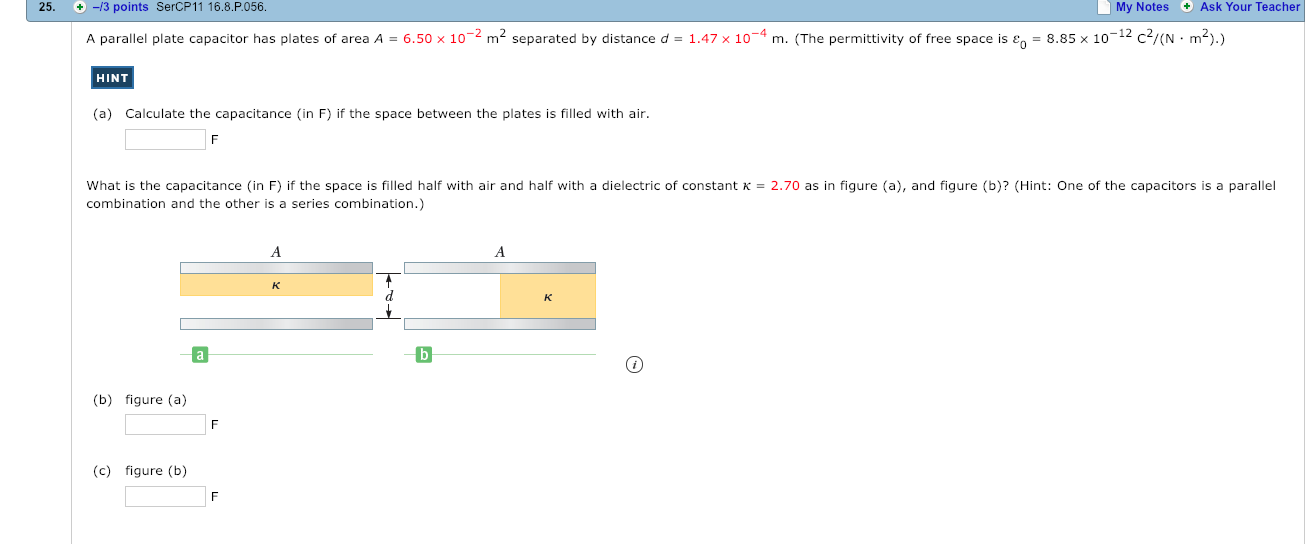# 25. -3points SercP11 16.8.P056.My Notes Ask Your TeacherA parallel plate capacitor has plates of area A-6.50 x 10-2 m2 separated by distance d-147x 10-4 m.The permitti ity of ree space ise885 x 10-12- N .HINT(a)Calculate the capacitance (in F) if the space between the plates is filled with airWhat is the capacitance (in F) if the space is filled half with air and half with a dielectric of constant K-2.70 as in figure (a), and figure (b)? (Hint: One of the capacitors is a parallelcombination and the other is a series combination.)(b) figure (a)(c) figure (b)

Question
9 viewshelp_outlineImage Transcriptionclose25. -3 points SercP11 16.8.P056. My Notes Ask Your Teacher A parallel plate capacitor has plates of area A-6.50 x 10-2 m2 separated by distance d-147x 10-4 m. The permitti ity of ree space ise 885 x 10-12- N . HINT (a) Calculate the capacitance (in F) if the space between the plates is filled with air What is the capacitance (in F) if the space is filled half with air and half with a dielectric of constant K-2.70 as in figure (a), and figure (b)? (Hint: One of the capacitors is a parallel combination and the other is a series combination.) (b) figure (a) (c) figure (b) fullscreen
check_circle

Step 1

The area of plates is 6.50 x 10-2 m2, the distance between the plates is 1.47 x 10-4 m, the permittivity of free space is 8.85 x 10-12 C2/(N.m2), the dielectric constant of air is 1.00 and the dielectric constant is 2.70.

(a)

In first case, the space in between the plates is filled with air. The expression for the capacitance of the capacitor is,

Step 2

Here, kair is the dielectric constant of air, A is the area of each plate, ε˳ is the permittivity of free space, d is the distance between the plates and C is the capacitance.

Thus, the capacitance of the capacitor is,

Step 3

(b)

In figure (a), the space in between the plates is half filled with air and half filled with a material which is parallel to the pla...

### Want to see the full answer?

See Solution

#### Want to see this answer and more?

Solutions are written by subject experts who are available 24/7. Questions are typically answered within 1 hour.*

See Solution
*Response times may vary by subject and question.
Tagged in

### Physics